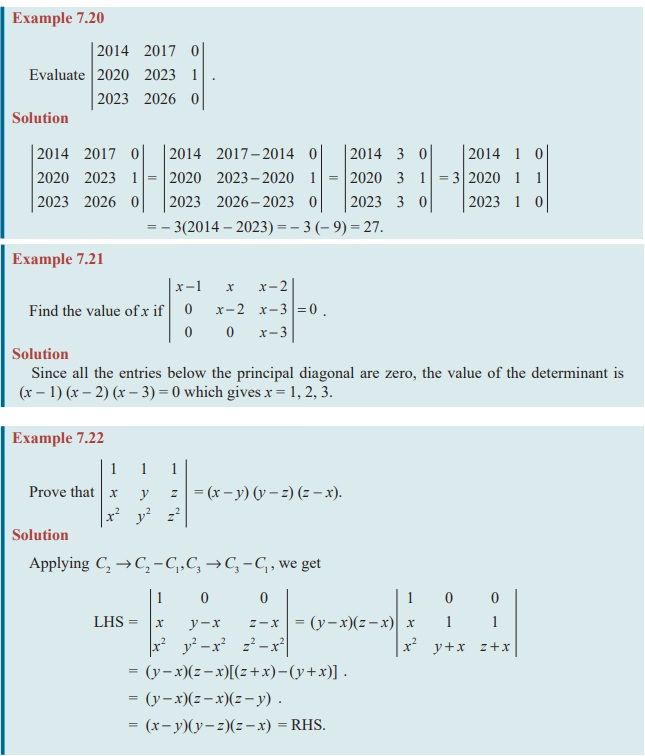Home | | Maths 11th std | Properties of Determinants

# Properties of Determinants

We can use one or more of the following properties of the determinants to simplify the evaluation of determinants.

Properties of Determinants

We can use one or more of the following properties of the determinants to simplify the evaluation of determinants.

### Property 1

The determinant of a matrix remains unaltered if its rows are changed into columns and columns into rows. That is, | A | = | AT | .

Since the row-wise expansion is same as the column-wise expansion, the result holds good.

### Property 2

If any two rows / columns of a determinant are interchanged, then the determinant changes in sign but its absolute value remains unaltered.

### Verification= a1 (b3 c2b2 c3 ) − b1 (a3 c2a2 c3 ) + c1 (a3 b2a2 b3 )

= − a1 (b2 c3b3 c2 ) + b1 (a2 c3a3 c2 ) − c1 (a2 b3a3 b2 )= −[ a1 (b2 c3b3 c2 ) − b1 (a2 c3a3 c2 ) + c1 (a2 b3a3b2 )]

= - | A |

Therefore, | A1 | = - | A | . Thus the property is verified.

Property 3

If there are n interchanges of rows (columns) of a matrix A then the determinant of the resulting matrix is (- 1)n | A |.

Property 4

If two rows (columns) of a matrix are identical, then its determinant is zero.

Verification

Let |A| =, with 2nd and 3rd rows are identical.

Interchanging second and third rows, we get − | A |  == | A |

2|A| = 0 |A| = 0.

Property 5

If a row (column) of a matrix A is a scalar multiple of another row (or column) of A, then its determinant is zero.

Note 7.8

(i) If all entries of a row or a column are zero, then the determinant is zero.

(ii) The determinant of a triangular matrix is obtained by the product of the principal diagonal elements.

Property 6

If each element in a row (or column) of a matrix is multiplied by a scalar k, then the determinant is multiplied by the same scalar k.

Verification= ka1 (b2 c3 b3 c2 ) kb1 (a2 c3 a3 c2 ) + kc1 (a2 b3 a3 b2 ) = k| A |

= k[a1 (b2 c3 b3 c2 ) b1 (a2 c3 a3 c2 ) + c1 (a2 b3 a3 b2 )] = k| A |

|A1| = k| A |.

Note 7.9

If A is a square matrix of order n, then

(i) | AB | = | A | | B |

(ii) If AB = O then either | A | = 0 or | B | = 0.

(iii) | An |= (| A |)n

Property 7

If each element of a row (or column) of a determinant is expressed as sum of two or more terms then the whole determinant is expressed as sum of two or more determinants.Verification

By taking first column expansion it can be verified easily.

LHS = (a1 + m1 )(b2 c3b3 c2 ) − ( a2 + m2 )(b1c3b3 c1 ) + ( a3 + m3 )(b1c2b2 c1 )

= a1 (b2 c3 b3 c2 ) a2 (b1c3 b3 c1 ) + a3 (b1c2 b2 c1 ) + m1 (b2 c3 b3 c2 ) m2 (b1c3 b3 c1 ) + m3 (b1c2b2 c1 )### Property 8

If, to each element of any row (column) of a determinant the equi-multiples of the corresponding entries of one or more rows (columns) are added or subtracted, then the value of the determinant remains unchanged.

### Verification| A1 | = | A | + p(0) + q(0) = | A | (using Property 4)

Therefore | A1 | = | A |

This property is independent of any fixed row or column.Study Material, Lecturing Notes, Assignment, Reference, Wiki description explanation, brief detail
11th Mathematics : UNIT 7 : Matrices and Determinants : Properties of Determinants |